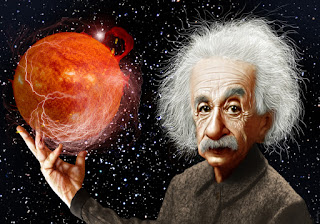19 September 2016

Find covariance $Cov(U^2,\sqrt{1-U^2})$
Let $U$ be uniform on $(0,1)$. Calculate $Cov(U^2,\sqrt{1-U^2})$. Also use simulation to approximate and then compare with the exact answer. Solution:
Using the traditional method will make it really hard. That's why we will use a trick to solve this problem. But first, from the definition we can write- \begin{align*} Cov(U^2,\sqrt{1-U^2})&= E(U^2,\sqrt{1-U^2})-E(U^2)E(\sqrt{1-U^2}) \end{align*} But... \begin{align*} E(U^2,\sqrt{1-U^2})-E(U^2)E(\sqrt{1-U^2})=\int_0^1 U^2\sqrt{1-U^2}dU\\-(\int_0^1 U^2 dU)(\int_0^1 \sqrt{1-U^2}dU) \end{align*} It's better but integral looks a little bit complex. Using traditional method to solve this integral will take long. So let's apply a trick..... Let, \begin{align} U&=sin\theta\\ dU&=cos\theta d\theta \\ \end{align} When $U\rightarrow 0$, $\theta\rightarrow 0$ and $U\rightarrow 1$, $\theta\rightarrow \pi/2$.
So our integration become \begin{align} I&=\int_0^{\pi/2} sin^2\theta cos^2\theta d\theta - \int_0^{\pi/2} sin^2\theta cos\theta d\theta \int_0^{\pi/2} cos^2\theta d\theta\\ &=\dfrac{1}{32}(4\theta-sin(4\theta))\bigg|_0^{\pi/2}-\dfrac{1}{3}sin^3\theta \bigg|_0^{\pi/2}(\dfrac{1}{2}(\theta+sin\theta cos\theta)\bigg|_0^{\pi/2}\\ &= \dfrac{\pi}{16}.\dfrac{1}{3}.\dfrac{\pi}{4} \end{align} So our final answer is - \begin{align} Cov(U^2,\sqrt{1-U^2})&=\dfrac{\pi^2}{192} \end{align}Physics Graduate and Data Science Enthusiast.